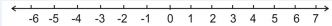### Number Systems - Revision Notes

CBSE Class 09 Mathematics

Revision Notes
CHAPTER – 1
NUMBER SYSTEMS

1 Rational Numbers

2 Irrational Numbers

3 Real Numbers and their Decimal Expansions

4 Operations on Real Numbers

5 Laws of Exponents for Real Numbers• Natural numbers are : 1, 2, 3, …………….. denoted by N.
• Whole numbers are : 0, 1, 2, 3, ……………… denoted by W.
• Integers : ……. -3, -2, -1, 0, 1, 2, 3, ……………… denoted by Z.
• Rational numbers - All the numbers which can be written in the form $\frac{p}{q}$ are called rational numbers where p and q are integers and $q\ne 0.$ Every integer p is also a rational number, can be written as $\frac{p}{1}.$
• Irrational numbers - A number is called irrational, if it cannot be written in the form $\frac{p}{q}$  where p and q are integers and $q\ne 0.$
• The decimal expansion of a rational number is either terminating or non terminating recurring. Thus we say that a number whose decimal expansion is either terminating or non terminating recurring is a rational number.
• Terminating decimals: The rational numbers with a finite decimal part or for which the long division terminates after a finite number of steps are known as finite or terminating decimals.
• Non-Terminating decimals: The rational numbers with an infinite decimal part or for which the long division does not terminate even after an infinite number of steps are known as infinite or non-terminating decimals
• The decimal expansion of a irrational number is non terminating non recurring.
• All the rational numbers and irrational numbers taken together make a collection of real numbers.
• A real number is either rational or irrational.
• If r is rational and s is irrational then r+s, r–s, r.s are always irrational numbers but $\frac{r}{s}$ may be rational or irrational.
• If n is a natural number other than a perfect square, then $\sqrt{n}$ is a irrational number.
• Negative of an irrational number is an irrational number.
• There is a real number corresponding to every point on the number line. Also, corresponding to every real number there is a point on the number line.
• Every irrational number can be represented on a number line using Pythagoras theorem.
• For every positive real number $x,$ $\sqrt{x}$ can be represented by a point on the number line by using the following steps:
1. Obtain all positive real numbers $x$ (say).
2. Draw a line and mark a point P on it.
3. Make a point Q on the line such that PQ = $x$ units.
4. From point Q marka distance of 1 unit and mark the new point as R.
5. Find the mid-point of PR and mark the point as O.
6. Draw a circle with centre O and radius OR.
7. Draw a line perpendicular to PR passing through Q and intersecting the semi-circle at S. Length QS is equal to $\sqrt{x}$.
• Rationalization means to remove square root from the denominator.

$\frac{3+\sqrt{5}}{\sqrt{2}}$ to remove we will multiply both numerator & denominator by $\sqrt{2}$

$\frac{1}{a±\sqrt{b}}$  its rationalization factor